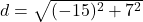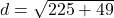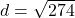## Find the distance using the modulus of the difference between z1 = -8 + 3i and z2 = 7 – 4i.

Question

Find the distance using the modulus of the difference between z1 = -8 + 3i and z2 = 7 – 4i.

in progress 0
3 weeks 2021-08-25T02:26:10+00:00 1 Answers 0 views 0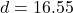Step-by-step explanation:

Given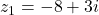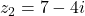Required

The distance between them

First, calculate the difference (d) between z1 and z2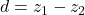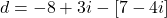Open bracket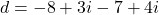Collect like terms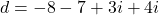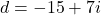Using modulus, we have: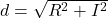Where

R = Real Part = -15

I = Imaginary Part = 7

So, we have: